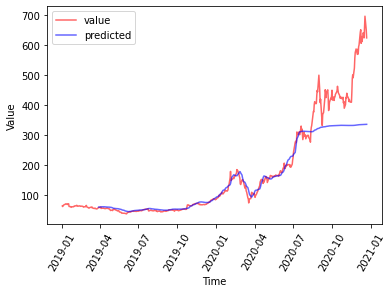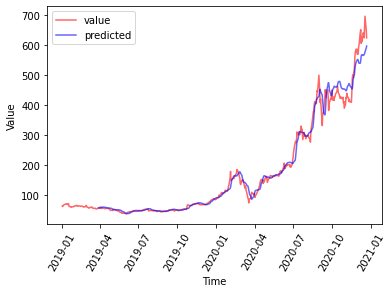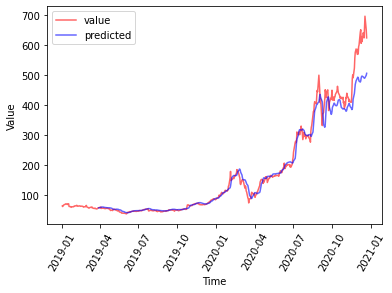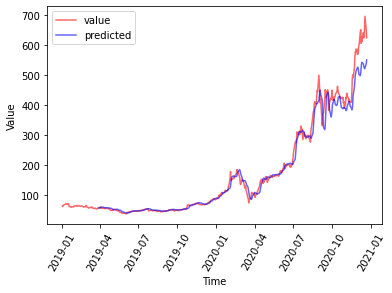There are many code samples on using LSTM networks for time series prediction. Rather than writing your own LSTM modules and gradient descent computations, usually we will use the off-the-shelf implementations from PyTorch or Tensorflow. Let’s look at a PyTorch model, from data preparation to evaluation:

import matplotlib.pyplot as plt
import numpy as np
import pandas as pd
import sklearn
import sklearn.preprocessing
import torch
import torch.nn as nn
import torch.optim as optim

NUM_EPOCHS = 100
WINSIZE = 60
BATCHSIZE = 32

# Read data, single column time series with datetime as index

N = len(df)
cutoff = int(N*0.8)

df_train = df.iloc[:cutoff][["ValueColumn"]]
scaler = sklearn.preprocessing.StandardScaler()
scaler.fit(df_train) # scaler needs 2D array as input, output is numpy array
timeseries = scaler.transform(df).astype('float32')

def sliding_window(a, win_size):
'''
Args:
a: numpy array of shape (N,M)
Returns:
numpy array of shape (K,w,M) where K=N-w+1
Modified from: https://stackoverflow.com/questions/6811183'
'''
shape = (a.shape - win_size + 1, win_size) + a.shape[-1:]
strides = (a.strides,) + a.strides
return np.lib.stride_tricks.as_strided(a, shape=shape, strides=strides)

class Model(nn.Module):
'LSTM neural network model in PyTorch'
def __init__(self, input_dim=1, hidden_dim=50, output_dim=1, num_layers=1, dropout=0.2):
super(Model, self).__init__()
self.input_dim = input_dim
self.hidden_dim = hidden_dim
self.output_dim = output_dim
# LSTM multilayer and dense layer
self.lstm = nn.LSTM(self.input_dim, self.hidden_dim, num_layers, dropout=dropout, batch_first=True)
self.dropout = nn.Dropout(p=dropout)
self.linear = nn.Linear(self.hidden_dim, self.output_dim)

def forward(self, input, hidden=None):
# input.shape = [batch_size, seq_length, input_dim]
# lstm_out.shape = [batch_size, seq_length, hidden_dim]
# self.hidden is a tuple of (h,c) which both are of shape [num_layers, batch_size, hidden_dim]
lstm_out, self.hidden = self.lstm(input, hidden)
# take output from the final timestep
y_pred = self.linear(self.dropout(lstm_out[:,-1,:]))
return y_pred # shape = [batch_size, out_dim]

'Batch dataset generator, expects a pair of (X,y) in numpy format'
X = torch.from_numpy(X)
y = torch.from_numpy(y)

# Prepare data: Sequences of fixed length
X = sliding_window(timeseries, WINSIZE)
Y = timeseries[WINSIZE:]
X = X[:len(Y)]
X_train = X[:cutoff-WINSIZE]
Y_train = Y[:cutoff-WINSIZE]

# Training, one batch per epoch
model = Model(input_dim=1, hidden_dim=50, output_dim=1, num_layers=4).float()
print(model)
loss_fn = torch.nn.MSELoss()
hist = []
for t in range(NUM_EPOCHS):
for i, (X_batch, y_batch) in enumerate(ldr):
y_pred = model(X_batch) # forward pass
loss = loss_fn(y_pred, y_batch.view(y_pred.shape))
hist.append(float(loss.item()))
loss.backward()
print("Epoch {:2s}: MSE = {}".format(t, hist[-1]))
torch.save(SAVEMODELFILE)

# Evaluation: Feed in the whole time series, then plot
X_pred = torch.from_numpy(X)
y_pred = model.eval()(X_pred).detach().numpy().reshape(-1,1)
y_pred = scaler.inverse_transform(y_pred)

plt.plot(df[['ValueColumn']], color='r', alpha=0.6, label='value')
plt.plot(df.index[WINSIZE:], y_pred, color='b', alpha=0.6, label='predicted')
plt.title('Time Series Prediction')
plt.xlabel('Time')
plt.ylabel('VALUE')
plt.tick_params(axis='x', labelrotation=60)
plt.legend()
plt.show()


and this is the equivalent in Tensorflow (2.x):

import matplotlib.pyplot as plt
import numpy as np
import pandas as pd
import sklearn
import sklearn.preprocessing
import tensorflow as tf

NUM_EPOCHS = 100
WINSIZE = 60
BATCHSIZE = 32

# Read data, single column time series with datetime as index

N = len(df)
cutoff = int(N*0.8)

df_train = df.iloc[:cutoff][["ValueColumn"]]
scaler = sklearn.preprocessing.StandardScaler()
scaler.fit(df_train) # scaler needs 2D array as input, output is numpy array
timeseries = scaler.transform(df).astype('float32')

def sliding_window(a, win_size):
'''
Args:
a: numpy array of shape (N,M)
Returns:
numpy array of shape (K,w,M) where K=N-w+1
Modified from: https://stackoverflow.com/questions/6811183'
'''
shape = (a.shape - win_size + 1, win_size) + a.shape[-1:]
strides = (a.strides,) + a.strides
return np.lib.stride_tricks.as_strided(a, shape=shape, strides=strides)

# LSTM neural network model in tensorflow.keras
dim_i, dim_h, dim_o = 1, 50, 1
p_dropout = 0.2
model = tf.keras.Sequential([
tf.keras.layers.LSTM(units=dim_h, return_sequences=True, input_shape=(winsize,dim_i)),
tf.keras.layers.Dropout(p_dropout),
tf.keras.layers.LSTM(units=dim_h, return_sequences=True),
tf.keras.layers.Dropout(p_dropout),
tf.keras.layers.LSTM(units=dim_h, return_sequences=True),
tf.keras.layers.Dropout(p_dropout),
tf.keras.layers.LSTM(units=dim_h), # no return_sequences at last LSTM stacking
tf.keras.layers.Dropout(p_dropout),
tf.keras.layers.Dense(units=dim_o) # regression, single output
])

# Prepare data: Sequences of fixed length
X = sliding_window(timeseries, WINSIZE)
Y = timeseries[WINSIZE:]
X = X[:len(Y)]
X_train = X[:cutoff-WINSIZE]
Y_train = Y[:cutoff-WINSIZE]

# Training, one batch per epoch
print(model.summary())
model.fit(X_train, Y_train, epochs=NUM_EPOCHS, batch_size=BATCHSIZE)
model.save(SAVEMODELFILE)

# Evaluation: Feed in the whole time series, then plot
y_pred = scaler.inverse_transform(model.predict(X))

plt.plot(df[['ValueColumn']], color='r', alpha=0.6, label='value')
plt.plot(df.index[WINSIZE:], y_pred, color='b', alpha=0.6, label='predicted')
plt.title('Time Series Prediction')
plt.xlabel('Time')
plt.ylabel('VALUE')
plt.tick_params(axis='x', labelrotation=60)
plt.legend()
plt.show()


Surely, PyTorch is slightly more verbose. These two snippet of code are making the same neural network models: Four LSTM layers and then a fully connected layer. Dropout layer interleaving each of them as a regularization mechanism. Each LSTM layer has hidden vector of dimension 50. The FC layer has single value as output. The network is trained using a window size of 60 of time series to predict the 61st value.

This is the result for the PyTorch network:and this is the result for the Tensorflow network:It is quite obvious that the result of PyTorch is less accurate than that of Tensorflow, despite all model construction parameters are the same. It is not a matter of the batch size, number of epochs, or the learning rate in the optimiser, as I tried to vary all of them. The reson that Tensorflow model can perform better then that of PyTorch consistently is because of the inner model of the LSTM layer. If we checked the doc, PyTorch is running a model of this:

\begin{aligned} i_t &= \sigma(W_{ii}x_t + b_{ii} + W_{hi}h_{t-1} + b_{hi}) \\ f_t &= \sigma(W_{if}x_t + b_{if} + W_{hf}h_{t-1} + b_{hf}) \\ g_t &= \tanh(W_{ig}x_t + b_{ig} + W_{hg}h_{t-1} + b_{hg}) \\ o_t &= \sigma(W_{io}x_t + b_{io} + W_{ho}h_{t-1} + b_{ho}) \\ c_t &= f_t \odot c_{t-1} + i_t \odot g_t \\ h_t &= o_t \odot \tanh(c_t) \end{aligned}

while Tensorflow does not lay out the equations in the doc, its model can be verified from the source code to be:

\begin{aligned} i_t &= \sigma(W_{ii}x_t + W_{hi}h_{t-1} + b_{i}) \\ f_t &= \sigma(W_{if}x_t + W_{hf}h_{t-1} + b_{f}) \\ g_t &= \tanh(W_{ig}x_t + W_{hg}h_{t-1} + b_{g}) \\ o_t &= \sigma(W_{io}x_t + W_{ho}h_{t-1} + b_{o}) \\ c_t &= f_t \odot c_{t-1} + i_t \odot g_t \\ h_t &= o_t \odot \tanh(c_t) \end{aligned}

The difference is in the linear transformation at each gate: PyTorch has two bias terms and Tensorflow has only one. Mathematically they are equivalent, because we can make $$b_i=b_{ii}+b_{hi}$$. In regression, however, this is a bad idea because PyTorch introduced extra degrees of freedom in the model and the extra parameters are collinear to othe parameters. This adversely affected the convergence and stability. To visualize the problem, consider what happened during gradient descent. Because $$b_i=b_{ii}+b_{hi}$$, it is easy to see that for any loss $$L$$, we have $$\partial L/\partial b_i = \partial L/\partial b_{ii} = \partial L/\partial b_{hi}$$. When we run optimiser.step() in the PyTorch model, both $$b_{ii}$$ and $$b_{hi}$$ are updated while updating only one of them should be enough, or updating both by half the amount.

From this we can explain why the PyTorch model has a higher error in general and horribly failed to predict the unseen validation data. We can indeed use no bias in LSTM layers by modifying the code into (PyTorch):

class Model(nn.Module):
'LSTM neural network model in PyTorch'
def __init__(self, input_dim=1, hidden_dim=50, output_dim=1, num_layers=1, dropout=0.2):
super(Model, self).__init__()
self.input_dim = input_dim
self.hidden_dim = hidden_dim
self.output_dim = output_dim
# LSTM multilayer and dense layer
self.lstm = nn.LSTM(self.input_dim, self.hidden_dim, num_layers, dropout=dropout, bias=False, batch_first=True)
self.dropout = nn.Dropout(p=dropout)
self.linear = nn.Linear(self.hidden_dim, self.output_dim)


and (Tensorflow):

model = tf.keras.Sequential([
tf.keras.layers.LSTM(units=dim_h, return_sequences=True, use_bias=False, input_shape=(winsize,dim_i)),
tf.keras.layers.Dropout(p_dropout),
tf.keras.layers.LSTM(units=dim_h, return_sequences=True, use_bias=False),
tf.keras.layers.Dropout(p_dropout),
tf.keras.layers.LSTM(units=dim_h, return_sequences=True, use_bias=False),
tf.keras.layers.Dropout(p_dropout),
tf.keras.layers.LSTM(units=dim_h, use_bias=False), # no return_sequences at last LSTM stacking
tf.keras.layers.Dropout(p_dropout),
tf.keras.layers.Dense(units=dim_o) # regression, single output
])


and the chart will be like this (PyToch):and this (Tensorflow):Without bias terms, the Tensorflow version will be worse. That is expected. However, PyTorch will perform better and produce a model closer than that of Tensorflow. This is yet another support to the argument that we should apply the simplest model in machine learning. Never overkill your data.